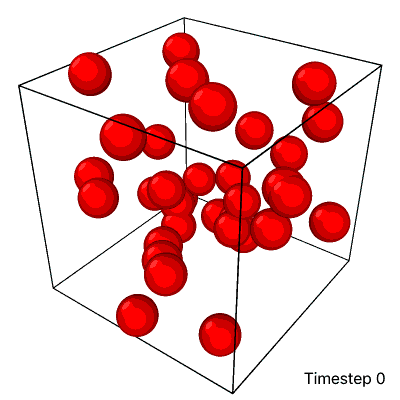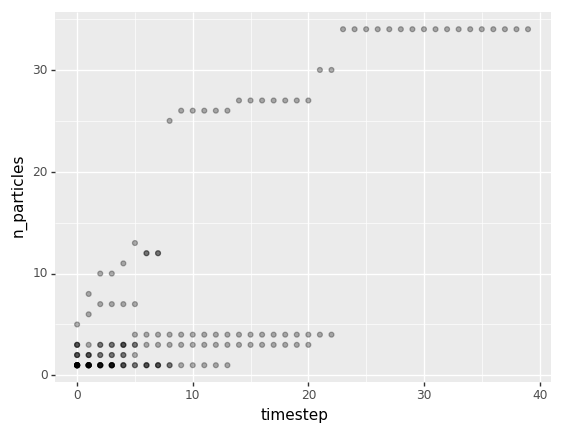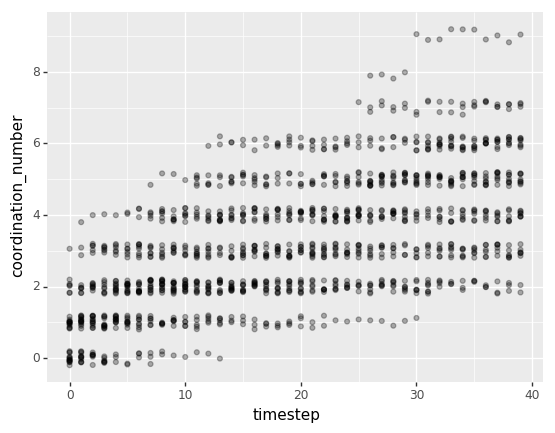# Example¶

In this example, we will analyze particle clustering by loading a .gro trajectory file (see here for details about this format), then passing that trajectory data to PBCluster. Your trajectories can come from wherever you want, but it’s your responsibility to get the particle coordinate data out of them to pass into PBCluster. That data needs to either be in the form of a 3D numpy array with shape (n_timesteps, n_particles, n_dimensions), or a pandas dataframe with columns for timestep, particle_id, x0, x1, and x2 (assuming a 3D simulation box).

Here’s a visualization made with Ovito of the particle trajectory we’ll be analyzing in this example:As the simulation progresses from timestep 0 to 39, the particles start dispersed and aggregate into a single cluster. At the end of this trajectory, it looks like there is a large cluster on the bottom and a separate, small cluster on top, but those are both part of the same cluster. Since this box has periodic boundaries (i.e. particles wrap around to the other side of the box if they pass through a face), the cluster wraps across the top and bottom faces of the box.

## Import Libraries¶

:

import mdtraj # for loading .gro data
import numpy as np
import pandas as pd
import plotnine as pn # for ggplot2-style plotting

from pbcluster import Trajectory


## Load .gro data¶

We need the particle coordinates and the simulation box size data.

:

traj_data = mdtraj.load("traj.gro")
# The factor of 10 is because .gro files store coordinates in Angstroms instead of nanometers
particle_coords = traj_data.xyz * 10
n_iterations, n_particles, n_dimensions = particle_coords.shape
print(f"n_iterations: {n_iterations}")
print(f"n_particles: {n_particles}")
print(f"n_dimensions: {n_dimensions}")

n_iterations: 40
n_particles: 34
n_dimensions: 3


So this trajectory file represents a 3D box of 34 particles moving around for 40 iterations.

Now let’s get the box lengths data:

:

box_lengths = traj_data.unitcell_lengths * 10
assert box_lengths.shape == (n_iterations, n_dimensions)
box_lengths[:5, :]

:

array([[25.33333, 25.33333, 25.33333],
[25.33333, 25.33333, 25.33333],
[25.33333, 25.33333, 25.33333],
[25.33333, 25.33333, 25.33333],
[25.33333, 25.33333, 25.33333]])


The PBCluster package requires the box size to stay the same for every iteration, so let’s double-check that that’s true and store just the first row in this array as the box lengths data.

:

assert np.allclose(box_lengths, box_lengths[0, 0])
box_lengths = box_lengths[0, :]
box_lengths

:

array([25.33333, 25.33333, 25.33333])


## Compute Cluster and Particle Properties¶

This package clusters particles based on a choice of a cutoff distance. Any 2 particles that are within that cutoff distance of each other will be part of the same cluster. The radial distribution function (calculated elsewhere) of this trajectory shows a first peak at $$r \approx 4.4$$, so I chose $$r = 4.95$$ to capture particle pairs in that peak.

:

cutoff_distance = 4.95
trajectory = Trajectory(particle_coords, box_lengths, cutoff_distance)


Let’s first calculate properties associated with particle clusters. In the dataframe below, each row represents a single cluster with an ID of cluster_id at timestep timestep.

:

cluster_properties_df = trajectory.compute_cluster_properties(properties="all")

:

asphericity center_of_mass_x0 center_of_mass_x1 center_of_mass_x2 cluster_id minimum_node_cuts_x0 minimum_node_cuts_x1 minimum_node_cuts_x2 n_particles rg timestep unwrapped_center_of_mass_x0 unwrapped_center_of_mass_x1 unwrapped_center_of_mass_x2
0 0.997920 2.973333 21.879999 1.625557 0 0 0 0 3 12.698203 0 2.973333 21.879999 26.958887
1 0.000000 16.469999 24.000000 12.610001 1 0 0 0 1 0.000000 0 16.469999 24.000000 12.610001
2 0.000000 10.280000 21.590000 8.280000 2 0 0 0 1 0.000000 0 10.280000 21.590000 8.280000
3 0.921179 12.858000 18.280000 4.704000 3 0 0 0 5 8.104199 0 12.858000 18.280000 4.704000
4 0.000000 13.049999 21.530001 15.700001 4 0 0 0 1 0.000000 0 13.049999 21.530001 15.700001

Now let’s calculate properties associated with individual particles. In the dataframe below, each row represents a single particle with an ID of particle_id at timestep timestep.

:

particle_properties_df = trajectory.compute_particle_properties(properties="all")

:

particle_id x0 x1 x2 coordination_number dx_from_com_x0 dx_from_com_x1 dx_from_com_x2 distance_from_com timestep cluster_id
0 0 2.150000 21.919998 22.560001 1 -0.823333 0.039999 -4.398886 4.475452 0 0
1 3 2.170000 21.500000 2.060000 2 -0.803333 -0.379999 0.434442 0.989184 0 0
2 25 4.600000 22.219999 5.590000 1 1.626667 0.340000 3.964443 4.298657 0 0
3 1 16.469999 24.000000 12.610001 0 0.000000 0.000000 0.000000 0.000000 0 1
4 2 10.280000 21.590000 8.280000 0 0.000000 0.000000 0.000000 0.000000 0 2

## Plot Cluster Properties¶

The following plot shows how the particle size distribution changes over time. Just like the rows in cluster_properties_df, each point represents a single cluster at a given iteration. I added some transparency to each point so overlapping points are seen as a little darker.

:

(
pn.ggplot(cluster_properties_df)
+ pn.geom_point(pn.aes(x='timestep', y='n_particles'), alpha=0.3)
):

<ggplot: (299276408)>


## Plot Particle Properties¶

The following plot shows how the coordination number distribution changes over time. Coordination number means the number of neighbors any given particle has. Here, just like the rows of particle_properties_df, each point represents a single particle at a given point in time. Since there are more total points in this chart, I added a little vertical jitter to each point to reduce the amount of overlap we see.

:

(
pn.ggplot(particle_properties_df)
+ pn.geom_jitter(pn.aes(x='timestep', y='coordination_number'), width=0, height=0.2, alpha=0.3)
+ pn.scale_y_continuous(breaks=[0, 2, 4, 6, 8])
):

<ggplot: (299356452)>


Overall, both of these plots corroborate what can be seen in the visualization at the top: that particles aggregate over time, forming larger and larger and more densely connected clusters until all particles in the box are part of a single cluster.